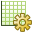# Specifying the Number Format for an Axis

Specify the number format for the axes and the number of significant digits that are display on the axes.

Select the graph where you want to change the major axis (or minor axis).
1. On the Cartesian context tab, on the Display tab, on the Axes group, click theAxis settings icon.
2. Select the axis that you want to modify.
• To modify the grid range for the horizontal axis, click Horizontal.
• To modify the grid range for the vertical axis, click Vertical.

Change the number format for the axis.

1. Under Number format, select one of the following:
1. From the Number format drop-down list, select one of the following:
• Decimal
• Scientific

Modify the number of significant digits for the axis.

1. Under Number format, select one of the following:
• To specify the number of significant digits that you want to see on the graph axis, clear the Automatically determine the number of significant digits check box.
• From the Number of significant digits drop-down list, select the number of digits you want to view on the graph.
• To determine the number of significant digits for the graph axis automatically, select the Automatically determine the number of significant digits check box.
2. Click OK to apply the settings and to close the dialog.